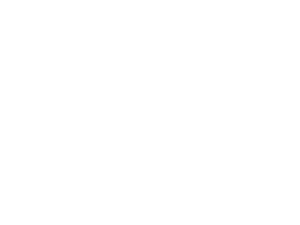# Important Formulas used in Mensuration

Mensuration is one of the topics in Quants. Now a days questions coming from this chapter also. Here we are providing you Some important formulas which is used in mensuration which are useful in SSC, Railways and other exams.Rectangle :

• Area = lb
• Perimeter  = 2(l+b)

Square :

• Area = a×a
• Perimeter = 4a

Parallelogram

• Area = l × h
• Perimeter = 2(l+b)

Triangle :

• Area =b×h/2 or √s(s-a)(s-b)(s-c)…………….where s=a+b+c/2

Right angle Triangle :

• Area =1/2(bh)
• Perimeter = b+h+d

Isosceles right angle triangle :

• Area = ½. a2
• Perimeter = 2a+d……………………….where d=a√2

Equilateral Triangle :

• Area = √3. a2/4 or  ½(ah)….where h = √3/2
• Perimeter = 3a

Trapezium :

• Area = 1/2h(a+b)
• Perimeter = Sum of all sides

Rhombus :

• Area = d1 × d2/2
• Perimeter = 4l

• Area =1/2 × Diagonal × (Sum of offsets)

Kite :

• Area  = d1×d2/2
• Perimeter  = 2 × Sum on non-adjacent sides

Circle :

• Area =  πr^2 or πd^2/4
• Circumference = 2πr  or πd
• Area of sector of a circle = (θπr^2 )/360

Frustum :

• Curved surface area = πh(r1+r2)
• Surface area = π( r12+ h(r1+r2) + r22)

Cube :

• Volume: V = l3
• Lateral surface area = 4a2
• Surface Area: S = 6s2
• Diagonal (d) = √3l

Cuboid :

• Volume of cuboid: lbh
• Total surface area = 2 (lb + bh + hl) or 6l2
• Length of diagonal =√(l^2+b^2+h^2)

Right Circular Cylinder :

• Volume of Cylinder = π r2 h
• Lateral Surface Area (LSA or CSA) = 2π r h
• Total Surface Area = TSA = 2 π r (r + h)
• Volume of hollow cylinder = π r h(R2 – r2)

Right Circular cone :

• Volume = 1/3 π r2h
• Curved surface area: CSA=  π r l
• Total surface area = TSA = πr(r + l )

Sphere

• Volume: V = 4/3 πr3
• Surface Area: S = 4πr2

Hemisphere :

• Volume = 2/3 π r3
• Curved surface area(CSA) = 2 π r2
• Total surface area = TSA = 3 π r2

Prism :

• Volume = Base area x h
• Lateral Surface area = perimeter of the base x h

Pyramid:

• Volume of a right pyramid = (1/3) × area of the base × height.
• Area of the lateral faces of a right pyramid = (1/2) × perimeter of the base x slant height.
• Area of whole surface of a right pyramid = area of the lateral faces + area of the base.

Tetrahedron :

• Area of its slant sides = 3a2√3/4
• Area of its whole surface = √3a2
• Volume of the tetrahedron = (√2/12) a 3

Regular Hexagon :

• Area =  3√3 a2 / 2
• Perimeter  = 6a

Some other Formula :

• Area of Pathway running across the middle of  a rectangle = w(l+b-w)
• Perimeter of Pathway around a rectangle field = 2(l+b+4w)
• Area of Pathway around a rectangle field =2w(l+b+2w)
• Perimeter of Pathway inside a rectangle field =2(l+b-4w)
• Area of Pathway inside a rectangle field =2w(l+b-2w)
• Area of four walls = 2h(l+b)

Note : If we missed anything please mention it, we will update it

AffairsCloud Recommends Oliveboard Mock Test

AffairsCloud Ebook - Support Us to Grow

error: Alert: Content is protected !!